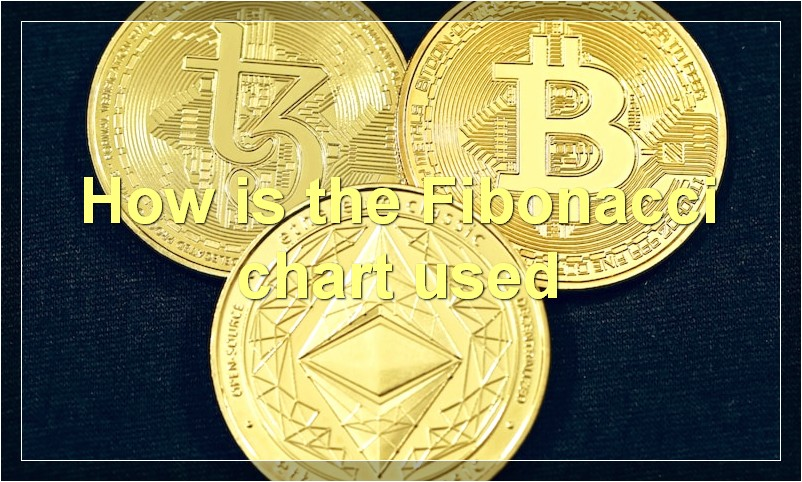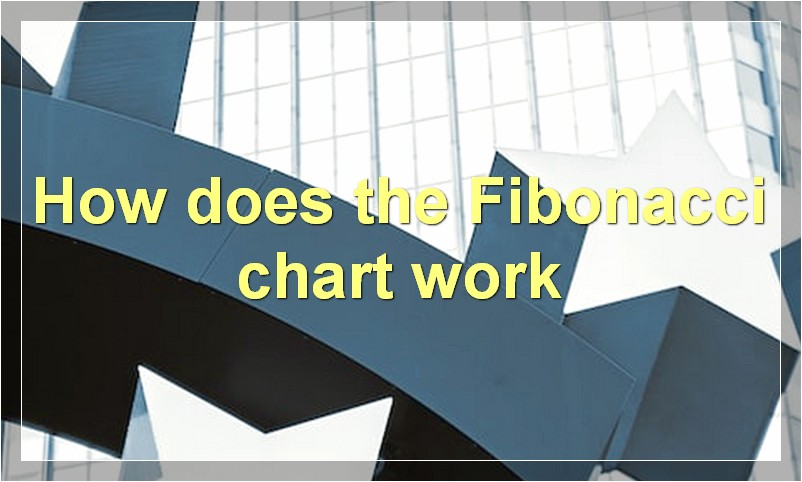# Fibonacci Charts: The Basics

If you’re looking to get started with Fibonacci charts, this is the article for you. We’ll cover the basics of what Fibonacci charts are and how to use them.

## What is the Fibonacci chart

In mathematics, the Fibonacci numbers or Fibonacci sequence are the numbers in the following integer sequence:

0, 1, 1, 2, 3, 5, 8, 13, 21, 34, 55, 89, 144…

By definition, the first two Fibonacci numbers are 0 and 1, and each subsequent number is the sum of the previous two.

The Fibonacci chart is a tool used by traders to predict future price movements based on past price movements. The theory behind the Fibonacci chart is that price moves in cycles and that these cycles can be predicted using the Fibonacci sequence.

The Fibonacci chart is created by plotting a series of vertical lines at key Fibonacci levels. These levels are derived from the Fibonacci sequence and are based on the premise that after a price move has completed, it will retrace a portion of that move before continuing in the original direction.

The key Fibonacci levels used in the Fibonacci chart are 23.6%, 38.2%, 50%, 61.8% and 100%. These levels are important because they represent potential support and resistance levels where price may find buyers or sellers and turn around.

Traders often use the Fibonacci chart in conjunction with other technical indicators to confirm price reversals. For example, if the Fibonacci chart is showing that price is likely to reverse at the 61.8% level and this level also lines up with a support or resistance level on another indicator, such as a moving average, then this could be considered a strong reversal signal.

The Fibonacci chart is a powerful tool that can be used to predict future price movements in the market. However, like all technical indicators, it should not be used alone but should be used in conjunction with other indicators to confirm signals.

## How is the Fibonacci chart usedThe Fibonacci chart is a technical analysis tool that is based on the Fibonacci sequence. The sequence is created by adding the previous two numbers together, starting with 0 and 1. The resulting numbers are then plotted on a graph, with the x-axis representing the time period and the y-axis representing the price.

The Fibonacci chart is used by traders to identify potential support and resistance levels, as well as trend reversals. The most important Fibonacci levels are 0.618 and 0.786, which represent the 61.8% and 78.6% retracements of a move, respectively. These levels are often used to set stop-loss orders and take-profit levels.

The Fibonacci chart can also be used to generate buy and sell signals. For example, a buy signal is generated when the price moves above the 61.8% Fibonacci level after a recent downtrend. Similarly, a sell signal is generated when the price moves below the 61.8% Fibonacci level after an uptrend.

## What are the benefits of using the Fibonacci chart

There are numerous benefits of using the Fibonacci chart when trading stocks. For one, the Fibonacci chart can help identify support and resistance levels. These levels are important because they can indicate where the stock price is likely to reverse course. By identifying these levels, traders can make informed decisions about when to buy or sell a stock.

Another benefit of using the Fibonacci chart is that it can help traders identify trendlines. Trendlines are important because they can show the overall direction of the market. By identifying a trendline, traders can make informed decisions about whether to buy or sell a stock.

Finally, the Fibonacci chart can also help traders identify entry and exit points. These points are important because they can help traders maximize their profits or minimize their losses. By identifying these points, traders can make informed decisions about when to enter or exit a trade.

## What is the history of the Fibonacci chart

The Fibonacci chart is a tool that was developed by the Italian mathematician Leonardo Fibonacci in the 13th century. The Fibonacci chart is used to help traders identify potential support and resistance levels in the market. The chart is based on the Fibonacci sequence, which is a series of numbers where each number is the sum of the previous two numbers. The Fibonacci sequence begins with 0, 1, 1, 2, 3, 5, 8, 13, 21, 34, 55, 89, 144, and so on. The Fibonacci chart is created by starting with the number 1 and then dividing it by the next number in the sequence (2). This produces a ratio of 1.5. The second number in the sequence (3) is then divided by the third number (5), which produces a ratio of 0.6. This process is continued until all the ratios in the Fibonacci sequence have been calculated. These ratios are then plotted on a graph.

## Who created the Fibonacci chart

In the Fibonacci chart, each number is the sum of the previous two. The first two numbers are 0 and 1, so the next number is 0 + 1 = 1, the next is 1 + 1 = 2, the next is 1 + 2 = 3, and so on.

Fibonacci was an Italian mathematician who lived in the 12th century. He is credited with introducing the Fibonacci sequence to the Western world.

## How does the Fibonacci chart workThe Fibonacci chart is a tool that traders use to help identify potential support and resistance levels in the market. The chart is based on the Fibonacci sequence, which is a series of numbers where each number is the sum of the two previous numbers. The Fibonacci sequence can be applied to any market, whether it’s stocks, commodities, or even Forex pairs.

The Fibonacci chart will have horizontal lines at certain Fibonacci ratios. These ratios are 23.6%, 38.2%, 50%, 61.8%, and 100%. The 23.6% and 38.2% lines are considered the most important because they represent the Golden Ratio, which is found throughout nature. The 50% line is considered important because it represents the halfway point between two extremes. The 61.8% line is important because it’s the inverse of the Golden Ratio, and the 100% line is important because it represents a complete reversal.

These lines are used as potential support and resistance levels because price often has a tendency to retrace to these levels after making a move. For example, if price moves up and then starts to retrace lower, traders will watch for the 23.6%, 38.2%, or 50% Fibonacci retracement levels as potential areas where price might find support and start moving higher again. Similarly, if price moves down and then starts to retrace higher, traders will watch for the 61.8% or 100% Fibonacci retracement levels as potential areas where price might find resistance and start moving lower again.

The Fibonacci chart is just one tool that traders can use to help them make decisions in the market. It’s important to remember that no tool is perfect, and that trader should always use a combination of different tools and techniques to make trading decisions.

## What are the applications of the Fibonacci chart

The Fibonacci chart is a mathematical tool that can be used in a variety of ways. One of the most popular applications is in technical analysis of financial markets. The Fibonacci chart can be used to predict support and resistance levels, as well as to identify trend reversals.

Another common application of the Fibonacci chart is in nature. The Fibonacci sequence can be found in the growth of many plant and animal species. For example, the petals of a flower often follow the Fibonacci sequence, as do the spirals of a seashell.

There are many other potential applications for the Fibonacci chart. Some people believe that it can be used to create more efficient algorithms, while others have proposed using it for everything from predicting traffic jams to finding extraterrestrial life.

Whatever its potential applications may be, the Fibonacci chart is an interesting tool that is definitely worth further exploration.

## What are some tips for using the Fibonacci chart

Assuming you want tips for investing:

The Fibonacci chart is a tool that can be used by investors to help make decisions about trading. There are a few things to keep in mind when using this tool. First, the Fibonacci chart is based on the Fibonacci sequence, which is a series of numbers that start with 0 and 1. The next number in the sequence is the sum of the previous two numbers. This sequence can be applied to trading by looking at the price of a security and determining how far it has moved up or down in relation to the previous day’s close. If the security has moved up by a Fibonacci number, it may be time to sell. Conversely, if the security has moved down by a Fibonacci number, it may be time to buy.

Another thing to keep in mind when using the Fibonacci chart is that not all securities will move in perfect Fibonacci patterns. However, many securities do tend to move in these patterns, so the Fibonacci chart can still be a helpful tool. Finally, it is important to remember that the Fibonacci chart is just one tool that can be used to make investment decisions. It should not be relied on exclusively, but rather used as one part of a larger investment strategy.

## What are some common mistakes people make when using the Fibonacci chart

When it comes to using the Fibonacci chart, there are a few common mistakes that people tend to make. First and foremost, people often fail to correctly align the Fibonacci sequence with the price action. This can lead to making inaccurate predictions about future price movements. Secondly, people often confuse Fibonacci retracements with Fibonacci extensions, which can again lead to incorrect predictions. Finally, many people incorrectly use Fibonacci Time Zones, which can result in them missing important price reversals.